The name “triangle” means, sensibly enough, “three angles” and indeed every triangle has three angles and three sides: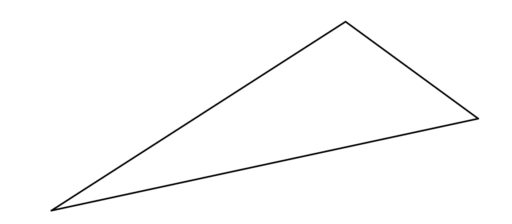This much is hopefully familiar. But just as with circles, there are many special terms people have devised for triangles. When it comes to the sides of a triangle, we can have:

Equilateral Triangles: All sides have the same length.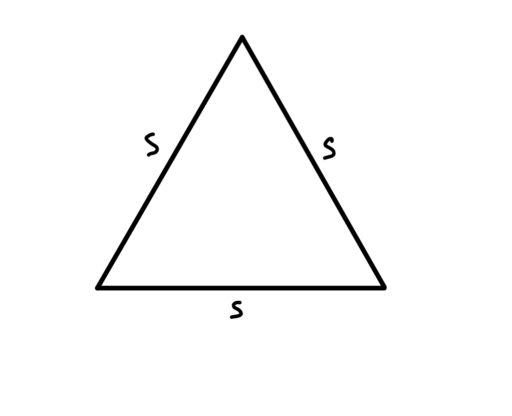Isosceles Triangles: Two sides have the same length as each other; the third has a different length.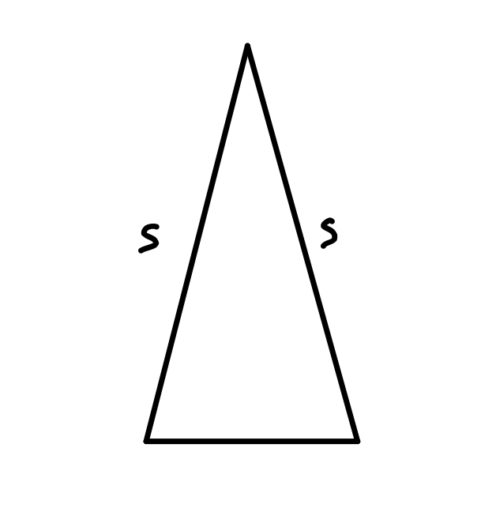Note that in an isosceles triangle:

Equal Sides Have Equal Angles: In an isosceles triangle, the angles opposite from the sides of equal length must have equal degree.

Thus, in the below diagram: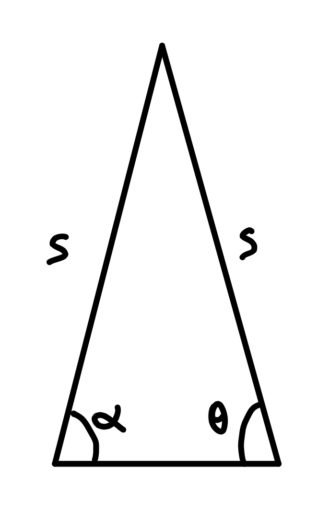anglesandare equal.

Scalene Triangles: All of the sides are of different lengths.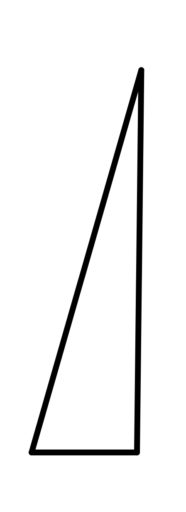We can also classify triangles according to their angles:

Acute Triangles: All angles are less than 90 degrees.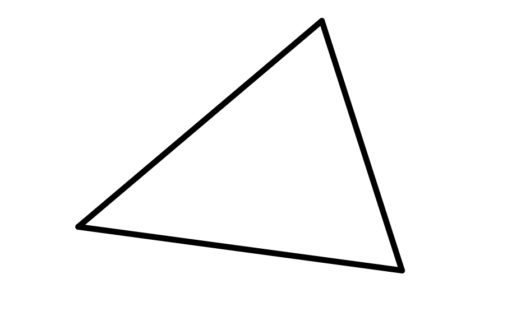Right Triangles: One angle is exactly 90 degrees.

We use a square to mark a 90 degree angle.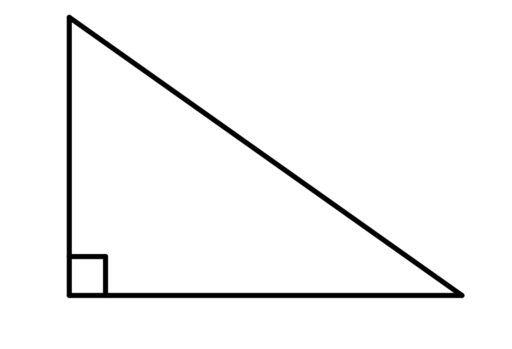Right triangles have lots of special further features which we will talk about here.

Obtuse Triangles: One angle is more than 90 degrees.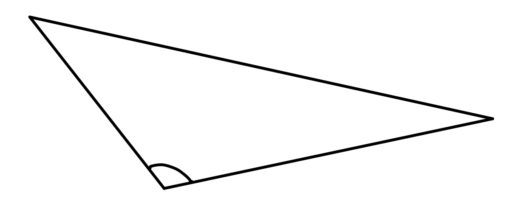Now, we can also compare two triangles to each other. We can say that they are:

Similar: Two triangles are similar if their angles have the same values.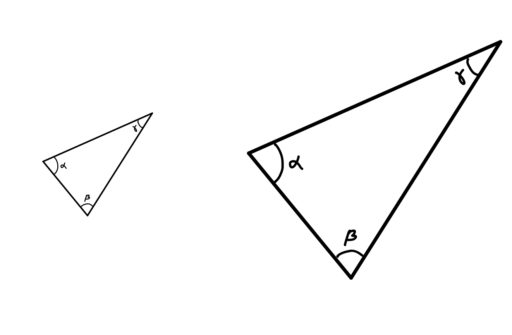So in the above diagram, even though one of the triangles is obviously bigger than the other, we can say that they are “similar” (in the technical, mathematical sense defined above) because their angles have the same values. And the definition makes sense since, after all, those two triangles do look pretty similar (in our ordinary, day-to-day sense)!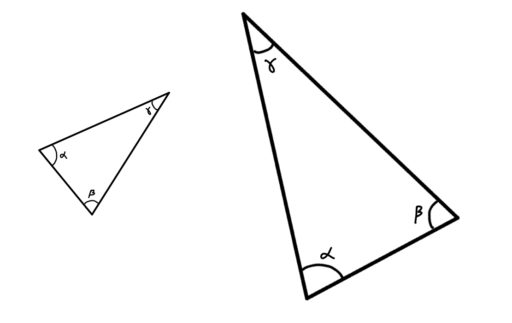These triangles are also similar to one another; it doesn’t matter if you rotate or flip the second triangle. All that matters is whether the angle values for the two figures are the same.

The reason why we care about the similarity of triangles is:

Similar Triangles Share Proportions: If two triangles are similar, then there is some constant ratio you can multiply the sides of one triangle by in order to get the sides of the other triangle.

For example, if the following two triangles are similar: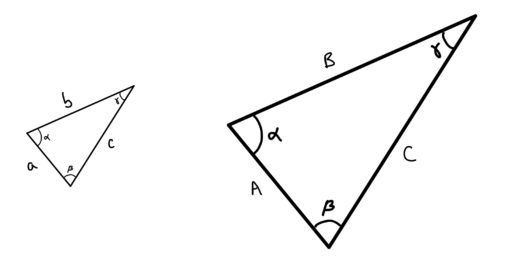then there is some constant, call it, such that:This can be very helpful in trying to find certain side lengths or the area of a triangle as we shall see.

Congruent: Two triangles are congruent if their angles are the same and their sides are the same length.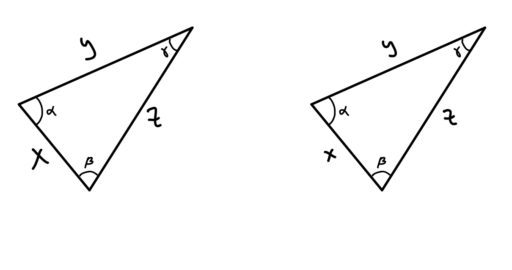You can think of congruence as signaling that those two triangles are effectively the same triangle. The lines are the same length, the angles are the same, the area is the same, and so on.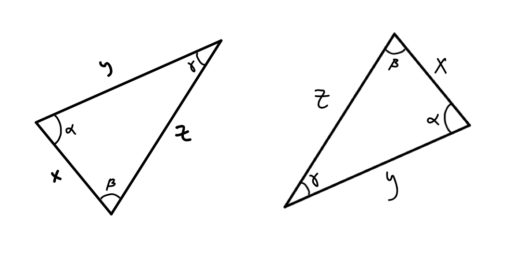And again, it doesn’t matter if you rotate or flip one of the triangles. It is still true that their angles and side lengths are the same, since rotating or flipping a shape won’t change any of that.

Now, when it comes to finding the area of a triangle, you need to know the base of the triangle and its height. The base can be any side of the triangle, but the height needs to extend perpendicular (at a right angle to) the base, and reach the highest point of the triangle, away from the base, like so: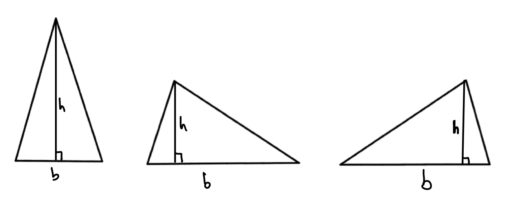Traditionally, we useto denote the base andto denote the height. Thus, we get:

Triangle Area Formula:Finally, we note two fundamental facts about triangles:

Angles of a Triangle Sum to 180: For any triangle, the sum of its interior angles is 180 degrees.

Triangle Inequality: The sum of the length of any two sides of a triangle is greater than the length of its third side.

Practice Problems:

1. Find the value of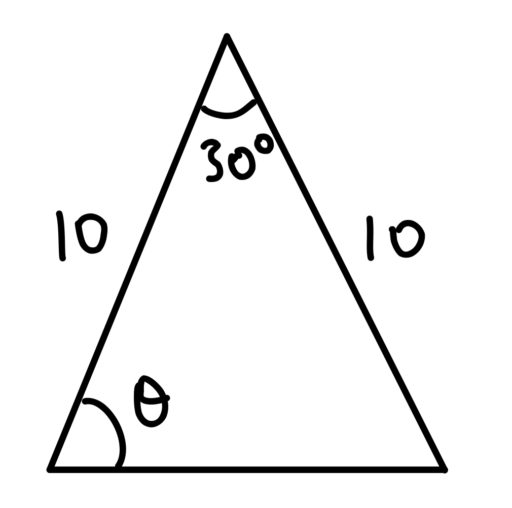2. Find the value of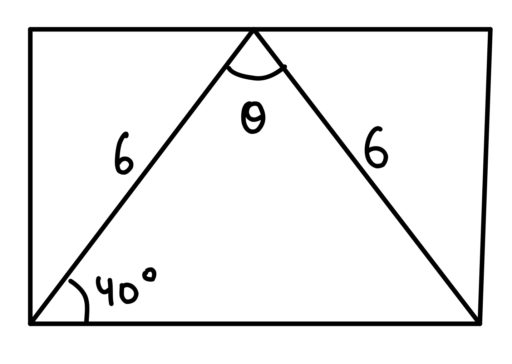3. A triangle has legs that areandunits long. What integer values are possible for the third side?